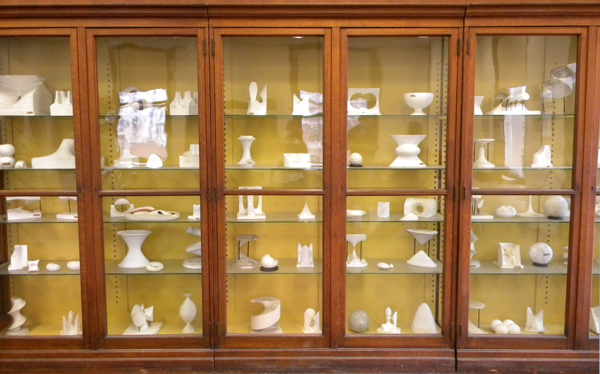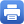Columbia Home
Algebraic GeometryThe objects of study of algebraic geometry are, roughly, the common zeroes of polynomials in one or several variables (algebraic varieties). But because polynomials are so ubiquitous in mathematics, algebraic geometry has always stood at the crossroads of many different fields. Classical questions in algebraic geometry involve the study of particular sets of equations or the geometry of lines and linear spaces. Among the kinds of questions that one can ask are enumerative ones: How many conics in the plane are tangent to a given set of five lines? How many lines are contained in a general surface of degree three in space? Another motivating question has been to try to fit together all algebraic varieties of a given type into a space which is itself an algebraic variety; such spaces are called moduli spaces. Simple examples of this type are projective spaces, which parameterize lines through the origin in a vector space, and their generalizations, Grassmannians, which parameterize linear subspaces of a vector space. In turn, the geometry of a Grassmannian can often be applied to solve an enumerative problem. In other kinds of moduli problems, one attempts to classify all curves, surfaces, or higher dimensional varieties of a certain type; another example is the space of all vector bundles of a given type over a fixed algebraic variety. Then one tries to construct and describe the moduli space of all such objects. Often invariant theory, i.e. the study of all invariant polynomials under the action of a group on a vector space, or a more general algebraic variety, plays a crucial role in the construction. In the attempt to answer these kinds of questions, algebraic geometry has moved from its classical beginnings to become a deep subject, drawing on a vast array of ideas in mathematics including commutative and homological algebra and category theory. The seminal figures in the development of modern algebraic geometry include Oscar Zariski, André Weil, Jean-Pierre Serre, and Alexandre Grothendieck.

Many properties of the zero sets of polynomials become most transparent when one considers solutions over the complex numbers. In this case, methods of topology, differential geometry, and partial differential equations can be applied. Recent developments in high energy physics have also led to a host of spectacular results and open problems in complex algebraic geometry. For example, the case where the dimension is one, i.e. the case of algebraic curves, is essentially the study of compact Riemann surfaces. This study has a long history involving calculus, complex analysis, and low dimensional topology. The moduli space of all compact Riemann surfaces has a very rich geometry and enumerative structure, which is an object of much current research, and has surprising connections with fields as diverse as geometric topology in dimensions two and three, nonlinear partial differential equations, and conformal field theory and string theory. Many questions posed by physicists have been solved by using the wealth of techniques developed by algebraic geometers. In turn, physics questions have led to new conjectures and new methods in this very central area of mathematics. For another example, the case of complex dimension two, i.e. of algebraic surfaces, has unexpected links to the study of four dimensional topology. Finally, the proposed ten dimensional space-time of string theory involves six very small extra dimensions, which correspond to certain three dimensional algebraic varieties, Calabi-Yau manifolds. These manifolds were already of great interest to mathematicians. Amazing ideas from physics have suggested that Calabi-Yau manifolds come in pairs. The geometry of the so-called mirror manifold of a Calabi-Yau manifold turns out to be connected to classical enumerative questions on the original manifold. In this way, for example, high energy physics was able to predict the number of lines (as well as more complicated curves) contained on a general hypersurface of dimension three and degree five.

Many questions in number theory concern the solutions of polynomials with integer coefficients over the integers or rational numbers, or modulo n for all natural numbers n, or over finite fields (which may be viewed as some kind of approximate solutions). Quite surprisingly, there is a striking interplay between the geometry of solutions over the complex numbers and number theory. Some of the spectacular recent developments in number theory, such as the solution of the Mordell conjecture (which is a statement about rational points on algebraic curves) or the role of elliptic and modular curves in the proof of Fermat’s last theorem, indicate the degree to which number theory and algebraic geometry are linked.

Finally, since polynomials lend themselves well to algebraic manipulation, there are many links between computational algebraic geometry and computer science. There are also surprising links to combinatorics through the theory of toric varieties.

The research group at Columbia University in algebraic geometry has a long tradition. In the sixties, Heisuke Hironaka’s fundamental work at Columbia on resolution of singularities of an algebraic variety was recognized with a Fields medal; twenty years later, Shigefumi Mori’s work on the classification of algebraic threefolds, also carried out at Columbia, was likewise so honored. Currently, the department is an active center of research in algebraic geometry with a strong geometric focus. Among the many areas of interest are the study of curves, surfaces, threefolds and vector bundles; geometric invariant theory; toric geometry; singularities; algebraic geometry in characteristic p and arithmetic algebraic geometry; connections between algebraic geometry and topology, mathematical physics, integrable systems, and differential geometry. Senior faculty in the algebraic geometry group at Columbia include Dave Bayer, Johan de Jong, Robert Friedman, Igor Krichever, Andrei Okounkov, Henry Pinkham, and Michael Thaddeus. The department also attracts many junior faculty and visitors, both senior and junior. Its graduate student and research seminars are a magnet for mathematicians throughout the New York area.Print this page